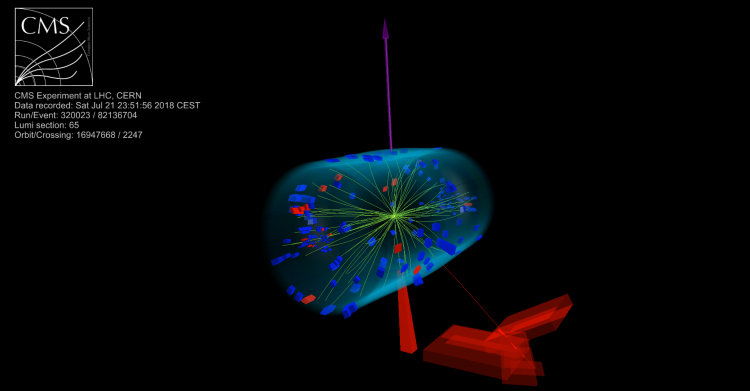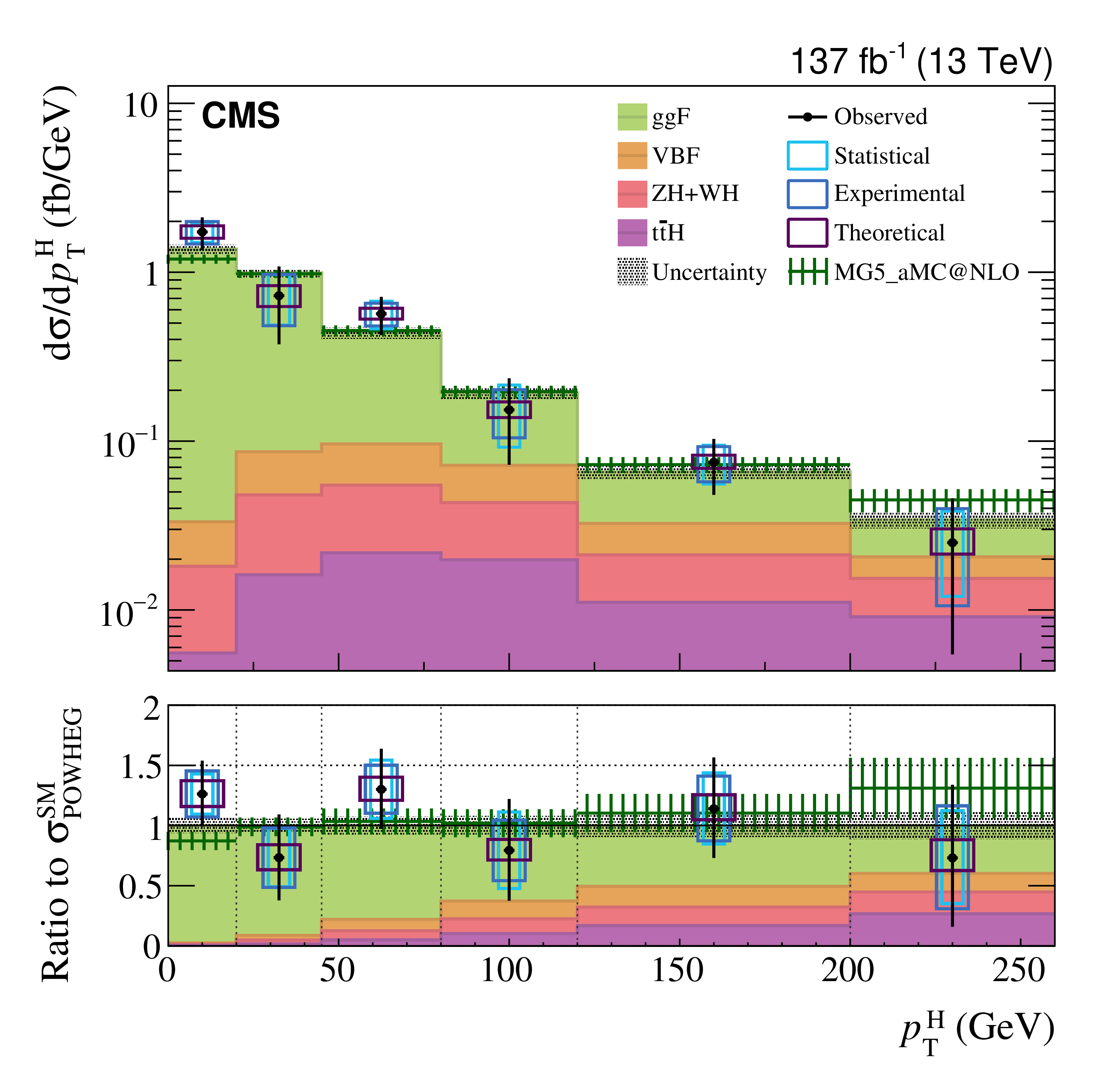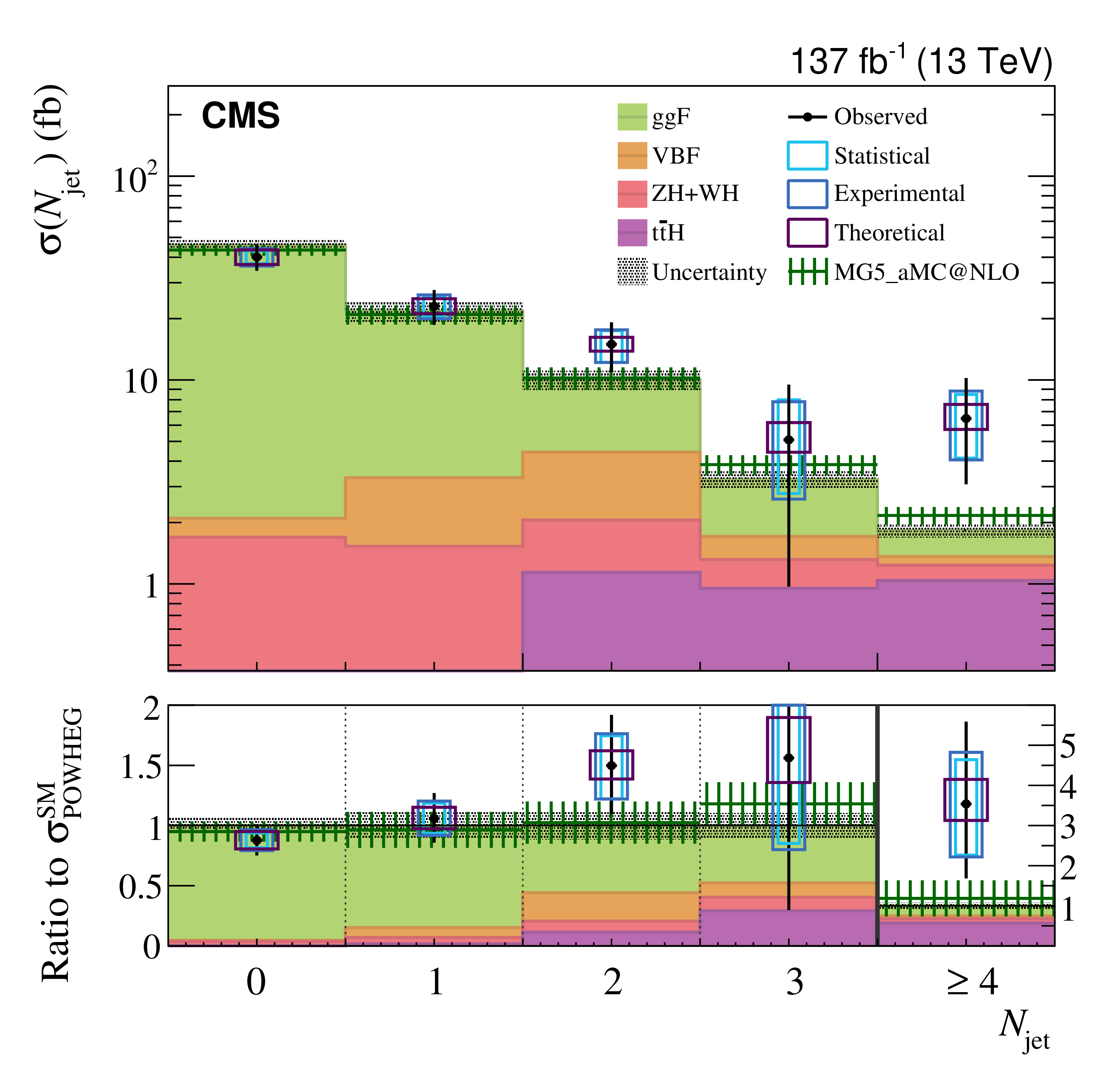•Each W boson in turn decays into a muon (red line pointing to the muon chambers shown in red) and a neutrino, and an electron (red tower) and a neutrino. The missing total momentum of the two neutrinos in the transverse plane is also measured (purple arrow).

In 2012 the CMS and ATLAS Collaborations announced the discovery of a new particle, that seems mostly consistent with the Higgs boson as predicted by the Standard Model. To understand if that is actually the case, physicists in the CMS collaboration dig deeper and measure the Higgs boson properties.

To understand the Higgs boson and its role in the Standard Model, we need to observe how it behaves. Does it prefer to be produced either with a small or large kinetic energy? Does the Higgs boson appear together with additional hadronic jet activity? This behaviour is intrinsically connected to how much the Higgs boson interacts with the other Standard Model particles or, in more scientific terms, the size of the Higgs boson coupling strength to other particles. Any significant change in behaviour compared to what we know from the Standard Model could imply that something beyond the Standard Model is playing a role. A precise measurement of the Higgs boson production properties is therefore crucial to have a thorough understanding of the Standard Model theory, but also represents a bridge towards the world of new physics and new particles.

The CMS Collaboration has recently exploited the full amount of data collected during the second run of the CERN LHC to probe the deepest secrets of the Higgs boson, measuring the additional energy and the number of extra particles produced together with Higgs bosons. Physicists do this by measuring the so-called differential production cross-section as a function of the Higgs boson transverse momentum, and the associated hadronic activity.

To understand how the Higgs particle behaves under those conditions, CMS physicists examined the case when the Higgs boson decays to a pair of W bosons. With a mass of about 80 GeV, the W boson is one of the particles that the Higgs boson prefers to interact with and decay to. After all, the Higgs boson ‘likes’ heavy particles, and the W boson mass is enough to make about one in five Higgs bosons decay to a pair of W bosons. W bosons can in turn decay to either hadrons or a charged lepton plus neutrino. The selection requires two charged leptons, either electrons or muons (with opposite charge), plus missing transverse momentum arising from the undetectable neutrinos. Collisions where both W bosons decay to leptons are easy to record by the CMS experiment, and have the additional advantage that the level of background collisions is well-understood.

Other Standard Model collisions create a background for the Higgs boson signal, and therefore must be reduced as much as possible to increase the precision of our measurement. An effective strategy is to reject the background using the known properties of the Higgs boson that the background does not show, for example, the mass of the Higgs particle itself. But in the case of two leptonic W boson decays, the presence of the neutrinos prevents reconstructing the full invariant mass of the Higgs boson. There are many alternatives to distinguish signal and backgrounds, for example exploiting the two charged leptons invariant mass or the transverse mass, including the missing transverse momentum information. One of the typical signatures of H→WW events is that the two charged leptons are produced close to each other in the detector, leading to rather small values of their invariant mass. On the other hand, for the main background processes, there is no such effect, and lepton directions are usually not correlated. All this information is finally combined to isolate a sample that is almost wholly consisting of Higgs bosons.

After the Higgs boson signal is isolated from the background, the next step is to measure the observables that most strikingly characterise the Higgs boson production: the transverse momentum spectrum ( pTH), and the associated number of hadronic jets (Njet). The  pTH shape is sensitive to how the Higgs boson couples with the light quarks, as well as to effects arising from higher-order effects in the Standard Model. The Njet shape instead is useful due to its close connection with the Higgs boson production mechanisms so those can be somewhat separated. The data are also corrected for the measurement uncertainty of the CMS detector; this technique is called unfolding. The two measured shapes with the background contributions subtracted are shown in Figure 1 and 2. The observed black data points are compared with the prediction of the Standard Model, showing the main Higgs boson production mechanisms in different colours.Figure 1: Higgs boson measured differential cross-section as a function of its transverse momentum. Black dots are the observed data. The coloured stacked histograms show the contribution of the main Higgs boson production mechanisms as predicted by the Standard Model. The coloured boxes represent the main uncertainty sources of this result.Figure 2: Higgs boson measured differential cross-section as a function of the number of associated jets. Black dots are the observed data. The coloured stacked histograms show the contribution of the main Higgs boson production mechanisms as predicted by the Standard Model. The coloured boxes represent the main uncertainty sources of this result.

There is no any significant deviation from what we would expect to see in the Standard Model. This measurement represents one of the most precise constraints of the pTH and Njet shapes ever done so far, but even so, the data still has large uncertainties.

In the third run of the LHC and after that the High-Luminosity LHC, the CMS experiment will collect even more data, which will help in improving the precision of the measurement, especially in the tails of the pTH and Njet distributions, which is the region where beyond the Standard Model effects could become sizable. But even now, thanks to this measurement, our knowledge of the Higgs boson is definitely getting in shape!